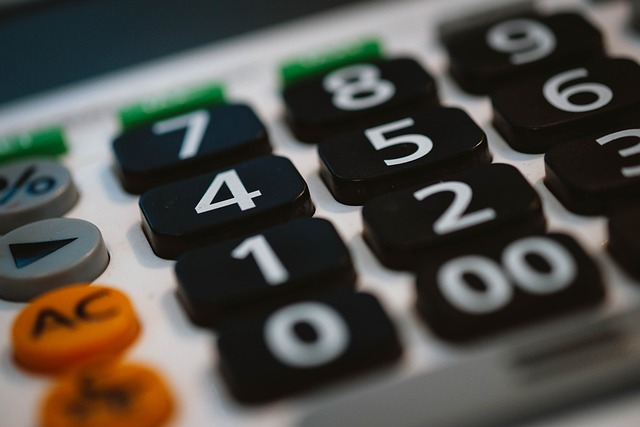^
You are here: HomeLessons → Calculator usage

# Should your child be allowed to use a calculator for math work?A calculator is a fantastic tool, but it can also do a lot of harm to children's learning of math. As parents and educators, we need to be careful about when we allow our students to use one.

I have received numerous comments on the harm that indiscriminate calculator usage can cause. If children are allowed to use calculators too early, and freely, their minds get “lazy,” and they will start relying on calculators even for simple things such as 6 × 7 or 320 + 50. It's just human nature!

The first years of elementary school is the time to build mental math skills and number sense — the ability to work with numbers flexibly — and a calculator therefore has almost no place in the math class during those years.

Number sense is more important than many people realize! It has been proven to predict a student's success in later years of math, including in algebra. For example, in one study1, researchers found that number sense in the beginning of first grade was strongly related to children's math abilities in 3rd grade.

As children work with numbers using mental math, they develop number sense. But using a calculator in K-3rd grade prevents this skill from developing! We all probably know or have heard of college students who don't know their multiplication tables. This seriously hinders them — and has already hindered them all through upper elementary school, middle school, and high school math by that point.

However, I realize that the calculator is useful. Students just need to learn when to use it.

In Math Mammoth materials, students are introduced to the calculator in 5th grade level, in a chapter dealing with large numbers. That chapter includes many problems where calculator usage is appropriate. For example, students practice estimating the result with mental math, and then using a calculator to find the exact answer. They also practice choosing whether mental math or a calculator is the best tool for a particular calculation.

These same lessons are also found in the Blue Series book Place Value 5.

From that point onward, Math Mammoth materials show a little calculator symbol next to the exercises where calculator usage is appropriate. That way, the curriculum gently guides them in using a calculator, while not hindering their mental math skills.

## What kind of calculator do I need for my child when using Math Mammoth?

For Math Mammoth grades 5-6, a very basic calculator will suffice. It needs to have the four basic operations. A phone or a computer calculator will do just fine. In fact, in a few exercises in grade 5, you will need to use a computer calculator that is able to display a large number of digits.

For 7th and 8th grades, the calculator will need to have the square root button.

### References & sources

(1) The Importance of Number Sense to Mathematics Achievement in First and Third Grades. Learn Individ Differ. 2010 Apr 1.

Number sense in infancy predicts mathematical abilities in childhood. PNAS. October 21, 2013.

Adolescents’ Functional Numeracy Is Predicted by Their School Entry Number System Knowledge. PLOS One, January 30, 2013

Number talks — short discussions among a teacher and students about how to solve a particular mental math problem. The focus is not on the correct answer, but on all the possible methods of finding the answer.

How to help students with multiplication tables?

Videos and games for memorizing addition & subtraction facts

By Maria Miller

WAIT!

Receive my monthly collection of math tips & resources directly in your inbox — and get a FREE Math Mammoth book!You can unsubscribe at any time.

### Math Mammoth Tour

Confused about the different options? Take a virtual email tour around Math Mammoth! You'll receive:

An initial email to download your GIFT of over 400 free worksheets and sample pages from my books. Six other "TOURSTOP" emails that explain the important things and commonly asked questions concerning Math Mammoth curriculum. (Find out the differences between all these different-colored series!)

This way, you'll have time to digest the information over one or two weeks, plus an opportunity to ask me personally about the curriculum.
A monthly collection of math teaching tips & Math Mammoth updates (unsubscribe any time)### "Mini" Math Teaching Course

This is a little "virtual" 2-week course, where you will receive emails on important topics on teaching math, including:

- How to help a student who is behind
- Troubles with word problems
- Teaching multiplication tables
- Why fractions are so difficult
- The value of mistakes
- Should you use timed tests
- And more!

A GIFT of over 400 free worksheets and sample pages from my books right in the very beginning.A monthly collection of math teaching tips & Math Mammoth updates (unsubscribe any time)
Enter your email to receive math teaching tips, resources, Math Mammoth news & sales, humor, and more! I tend to send out these tips about once monthly, near the beginning of the month, but occasionally you may hear from me twice per month (and sometimes less often).• A GIFT of over 400 free worksheets and sample pages from my books.